# NCERT Exemplar - Linear Programming Notes | EduRev

## JEE : NCERT Exemplar - Linear Programming Notes | EduRev

The document NCERT Exemplar - Linear Programming Notes | EduRev is a part of the JEE Course Mathematics (Maths) Class 12.
All you need of JEE at this link: JEE

Q.1. Determine the maximum value of Z = 11x + 7y subject to the constraints : 2x + y ≤ 6, x ≤ 2, x ≥ 0, y ≥ 0.
Ans.
Given that: Z = 11x + 7y and the constraints 2x + y ≤ 6, x ≤ 2, x ≥ 0, y ≥ 0.
Let 2x + y = 6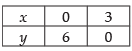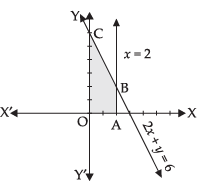The shaded area OABC is the feasible region determined by the constraints
2x + y ≤ 6, x ≤ 2, x ≥ 0, y ≥ 0.
The feasible region is bounded.
So, maximum value will occur at a corner point of the feasible region.
Corner points are (0, 0), (2, 0), (2, 2) and (0, 6).
Now, evaluating the value of Z, we get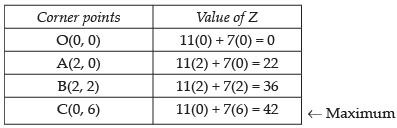Hence, the maximum value of Z is 42 at (0, 6).

Q.2. Maximise Z = 3x + 4y, subject to the constraints: x + y ≤ 1, x ≥ 0, y ≥ 0.
Ans.
Given that: Z = 3x + 4y and the constraints x + y ≤ 1, x ≥ 0, y ≥ 0.
Let x + y = 1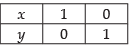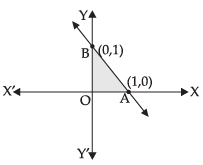The shaded area OAB is the feasible region determined by
x + y ≤ 1, x ≥ 0, y ≥ 0.
The feasible region is bounded.
So, maximum value will occur at the corner points O(0, 0), A(1, 0), B(0, 1).
Now, evaluating the value of Z, we get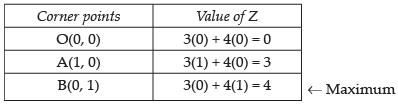Hence, the maximum value of Z is 4 at (0, 1).

Q.3. Maximise the function Z = 11x + 7y, subject to the constraints: x ≤ 3, y ≤ 2, x ≥ 0, y ≥ 0.
Ans.
The shaded region is the feasible region determined by the constraints x ≤ 3, y ≤ 2, x ≥ 0, y ≥ 0.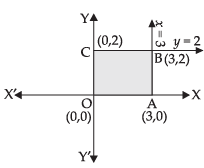The feasible region is bounded with four corners O(0, 0), A(3, 0), B(3, 2) and C(0, 2).
So, the maximum value can occur at any corner.
Let us evaluate the value of Z.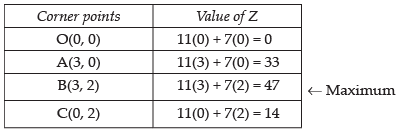Hence, the maximum value of the function Z is 47 at (3, 2).

Q.4. Minimise Z = 13x – 15y subject to the constraints : x + y ≤ 7, 2x – 3y + 6 ≥ 0, x ≥ 0, y ≥ 0.
Ans.
Given that: Z = 13x – 15y and the constraints
x + y ≤ 7, 2x – 3y + 6 ≥ 0, x ≥ 0, y ≥ 0.
Let x  + y = 7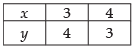Let 2x – 3y + 6 = 0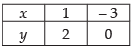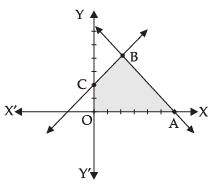The shaded region is the feasible region determined by the constraints
x + y ≤ 7, 2x – 3y + 6 ≥ 0, x ≥ 0, y ≥ 0.
The feasible region is bounded with four corners O(0, 0), A(7, 0), B(3, 4), C(0, 2)
So, the maximum value can occur at any corner.
Let us evaluate the value of Z.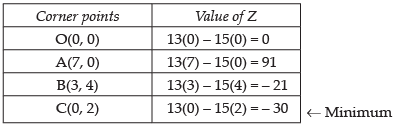Hence, the minimum value of Z is – 30 at (0, 2).

Q.5. Determine the maximum value of Z = 3x + 4y if the feasible region (shaded) for a LPP is shown in Figure.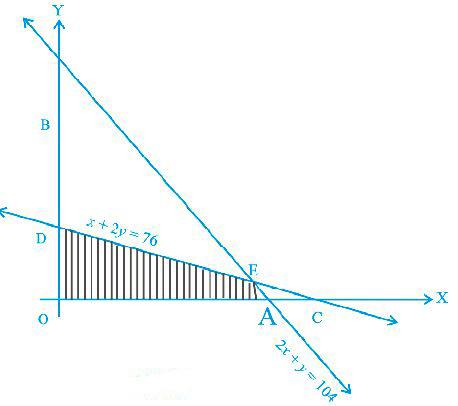Ans.
As shown in the figure, OAED is the feasible region.
At A, y = 0
∴ 2x + y = 104
⇒ x = 52
Which gives corner point A = (52, 0)
At D, x = 0 ∴ x + 2y = 76
⇒ y = 38
Which gives corner point D = (0, 38)
Now solving the given equations, we get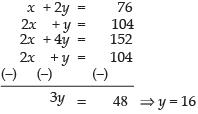x + 2(16) = 76
⇒ x = 76 – 32 = 44
So, the corner point E = (44, 16)
Evaluating the maximum value of Z, we get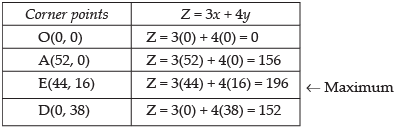Hence, the maximum value of Z is 196 at (44, 16).

Q.6. Feasible region (shaded) for a LPP is shown in Figure. Maximise Z = 5x + 7y.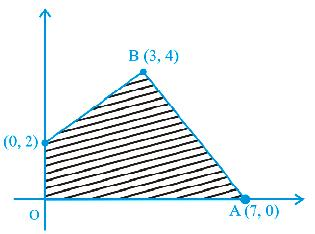Ans.
OABC is the feasible region whose corner points are O(0, 0), A(7, 0), B(3, 4) and C(0, 2)
Evaluating the value of Z, we get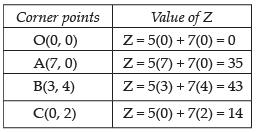← Maximum
Hence, the maximum value of Z is 43 at (3, 4).

Q.7. The feasible region for a LPP is shown in Figure. Find the minimum value of Z = 11x + 7y.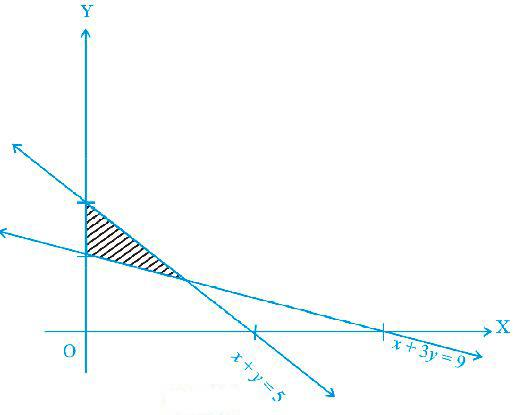Ans.
As per the given figure, ABCA is the feasible region.
Corner points C(0, 3), B(0, 5) and for A,
we have to solve equations
x + 3y = 9
and x + y = 5
Which gives x = 3, y = 2
i.e., A(3, 2)
Evaluating the value of Z, we get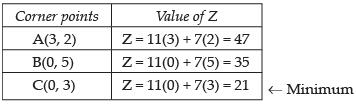Hence, the minimum value of Z is 21 at (0, 3).

Q.8. Refer to Exercise 7 above. Find the maximum value of Z.
Ans.
As per the evaluating table for the value of Z, it is clear that the maximum value of Z is 47 at (3, 2).

Q.9. The feasible region for a LPP is shown in Figure. Evaluate Z = 4x + y at each of the corner points of this region. Find the minimum value of Z, if it exists.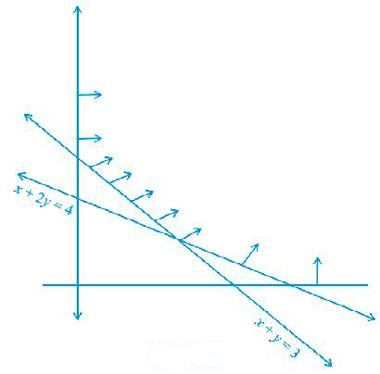Ans.
As per the given figure, ABC is the feasible region which is open unbounded.
Here, we have
x + y = 3 ...(i)
and x + 2y = 4 ...(ii)
Z = 4x + y
Solving eq. (i) and (ii), we get
x = 2 and y = 1
So, the corner points are
A(4, 0), B(2, 1) and C(0, 3)
Let us evaluate the value of Z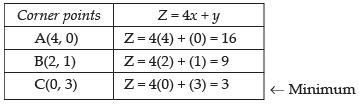Now, the minimum value of Z is 3 at (0, 3) but since, the feasible region is open bounded so it may or may not be the minimum value of Z.
Therefore, to face such situation, we draw a graph of 4x + y < 3 and check whether the resulting open half plane has no point in common with feasible region. Otherwise Z will have no minimum value. From the graph, we conclude that there is no common point with the feasible region.
Hence, Z has the minimum value 3 at (0, 3).

Q.10. In Figure, the feasible region (shaded) for a LPP is shown. Determine the maximum and minimum value of Z = x + 2y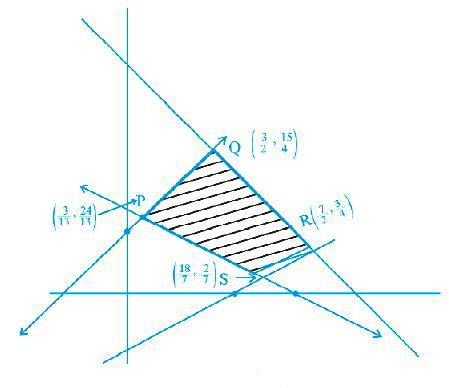Ans.
Here, corner points are given as follows: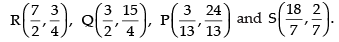Now, evaluating the value of Z for the feasible region RQPS.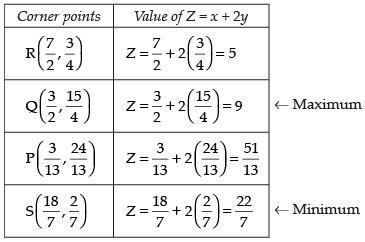Hence, the maximum value of Z is 9 at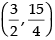and the minimum value of Z is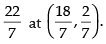Q.11. A manufacturer of electronic circuits has a stock of 200 resistors, 120 transistors and 150 capacitors and is required to produce two types of circuits A and B. Type A requires 20 resistors, 10 transistors and 10 capacitors. Type B requires 10 resistors, 20 transistors and 30 capacitors. If the profit on type A circuit is Rs 50 and that on type B circuit is Rs 60, formulate this problem as a LPP so that the manufacturer can maximise his profit.
Ans.
Let x  units of type A and y units of type B electric circuits be produced by the manufacturer.
As per the given information, we construct the following table: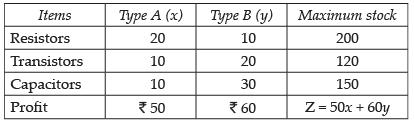Now, we have the total profit in rupees Z = 50x + 60y  to maximise subject to the constraints
20x + 10y ≤ 200  ...(i);
10x + 20y ≤ 120 ...(ii)
10x + 30y ≤ 150 ...(iii);
x ≤ 0, y ≤ 0 ...(iv)
Hence, the required LPP is
Maximise Z = 50x + 60y subject to the constraints
20x + 10y ≤ 200
⇒ 2x + y ≤ 20;  10x + 20y ≤ 120
⇒ x + 2y ≤ 12
and 10x + 30y ≤ 150
⇒ x + 3y ≤ 15, x ≤ 0, y ≤ 0

Q.12. A firm has to transport 1200 packages using large vans which can carry 200 packages each and small vans which can take 80 packages each. The cost for engaging each large van is Rs 400 and each small van is Rs 200. Not more than Rs 3000 is to be spent on the job and the number of large vans can not exceed the number of small vans. Formulate this problem as a LPP given that the objective is to minimise cost.
Ans.
Let x  and y be the number of large and small vans respectively. From the given information, we construct the following corresponding constraints table;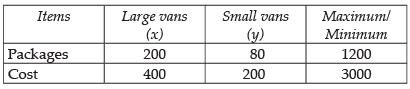Now the objective function for minimum cost is
Z = 400x + 200y
Subject to the constraints;
200x + 80y ≥ 1200 ⇒ 5x + 2y ≥ 30 ...(i)
400x + 200y ≤ 3000 ⇒ 2x + y ≤ 15 ...(ii)
x ≤ y...(iii)
and x ≥ 0, y ≥ 0 (non-negative constraints)
Hence, the required LPP is to minimise Z = 400x + 200y
Subject to the constraints 5x + 2y ≥ 30, 2x + y ≤ 15, x ≤ y and x ≥ 0, y ≥ 0.

Q.13. A company manufactures two types of screws A and B. All the screws have to pass through a threading machine and a slotting machine. A box of Type A screws requires 2 minutes on the threading machine and 3 minutes on the slotting machine. A box of type B screws requires 8 minutes of threading on the threading machine and 2 minutes on the slotting machine. In a week, each machine is available for 60 hours.
On selling these screws, the company gets a profit of Rs 100 per box on type A screws and Rs 170 per box on type B screws.
Formulate this problem as a LPP given that the objective is to maximise profit.
Ans.
Let the company manufactures x boxes of type A screws and y boxes of type B screws.
From the given information, we can construct the following table.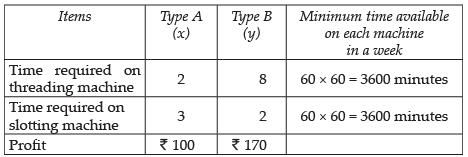As per the information in the above table, the objective
function for maximum profit Z = 100x + 170y
Subject to the constraints
2x + 8y ≤ 3600
⇒ x + 4y ≥ 1800   ...(i)
3x + 2y ≥ 3600   ...(ii)
x ≤ 0, y ≤ 0 (non-negative constraints)
Hence, the required LPP is Maximise Z = 100x + 170y
Subject to the constraints,
x + 4y ≥ 1800, 3x + 2y ≥ 3600, x ≤ 0, y ≤ 0.

Q.14. A company manufactures two types of sweaters : type A and type B. It costs Rs 360 to make a type A sweater and Rs 120 to make a type B sweater. The company can make at most 300 sweaters and spend at most Rs 72000 a day.
The number of sweaters of type B cannot exceed the number of sweaters of type A by more than 100. The company makes a profit of Rs 200 for each sweater of type A and Rs 120 for every sweater of type B.
Formulate this problem as a LPP to maximise the profit to the company.
Ans.
Let x  and y be the number of sweaters of type A and type B respectively.
From the given information, we have the following constraints.
360x + 120y ≤ 72000 ⇒ 3x + y ≤ 600 ...(i)
x + y ≤ 300     ...(ii);
x + 100 ≥ y ⇒ y ≤ x + 100 ...(iii)
Profit (Z) = 200x + 120y
Hence, the required LPP to maximise the profit is
Maximise Z = 200x + 120y
subject to the constraints
3x + y ≤ 600, x + y ≤ 300, y ≤ x + 100, x ≥ 0, y ≥ 0.

Q.15. A man rides his motorcycle at the speed of 50 km/hour. He has to spend Rs 2 per km on petrol. If he rides it at a faster speed of 80 km/hour, the petrol cost increases to Rs 3 per km. He has atmost Rs 120 to spend on petrol and one hour’s time. He wishes to find the maximum distance that he can travel.
Express this problem as a linear programming problem.
Ans.
Let the man covers x km on his motorcycle at the speed of 50 km/hr and covers y km at the speed of 80 km/hr.
So, cost of petrol = 2x + 3y
The man has to spend ₹ 120 atmost on petrol
∴ 2x + 3y ≤ 120 ...(i)
Now, the man has only 1 hr time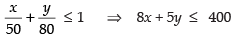x ³ 0, y ³ 0
To have maximum distance Z = x + y.
Hence, the required LPP to travel maximum distance is maximise Z = x + y, subject to the constraints
2x + 3y ≤ 120, 8x + 5y ≤ 400, x ≥ 0, y ≥ 0.

Q.16. Refer to Exercise 11. How many of circuits of Type A and of Type B, should be produced by the manufacturer so as to maximise his profit? Determine the maximum profit.
Ans.
As per the solution of Question No. 11, we have
Maximise Z = 50x + 60y subject to the contraints
2x + y ≤ 20 ...(i)
x + 2y ≤ 12 ...(ii)
x + 3y ≤ 15 ...(iii)
x ≥ 0, y ≥ 0...(iv)
Let us draw the table for the above statements
Table for (i)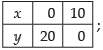Table for (ii)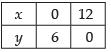Table for (iii)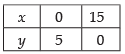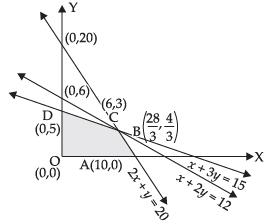Solving eq. (i) and (ii) we get,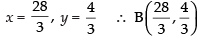is the corner
Solving eq. (ii) and (iii) we get,
x = 6, y = 3
∴ C(6, 3) is the corner
Solving eq. (i) and (iii) we get,
x = 9, y = 2 (not included in the feasible region)
Here, OABCD is the feasible region.
So, the corner points are O(0, 0), A(10, 0),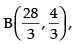C(6, 3) and D(0, 5).
Let us evaluate the value of Z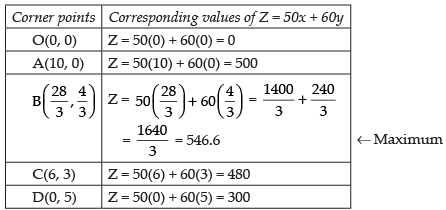Here, the maximum profit is ₹ 546.6 which is not possible for number of items in fraction.
Hence, the maximum profit for the manufacturer is ₹ 480 at (6, 3). Type A = 6 and Type B = 3.

Q.17. Refer to Exercise 12. What will be the minimum cost?
Ans.
As per the solution of Q. 12., we have Z = 400x + 200y
Subject to the constraints
5x + 2y ≥ 30 ...(i)
2x + y ≤ 15 ...(ii)
x ≤ y, x ≥ 0, y ≥ 0
x - y ≤ 0  ...(iii)
Let 5x + 2y = 30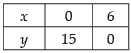Let 2x + y = 15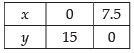Let x  – y = 0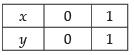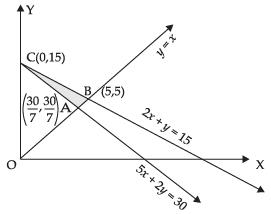Solving eq. (i) and (iii) we get;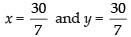and on solving eq. (ii) and (iii) we get, x = 5 and y = 5
Here, ABC is the shaded feasible region whose corner points are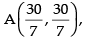B(5, 5) and C(0, 15)
Evaluating the value of Z, we have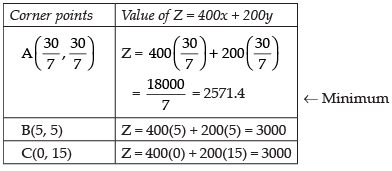Hence, the required minimum cost is ₹ 2571.4 at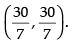Q.18. Refer to Exercise 13. Solve the linear programming problem and determine the maximum profit to the manufacturer.
Ans.
As per the solution of Q. 13, we have:
Let 3x + 2y = 3600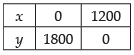Let x  + 4y = 1800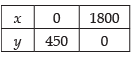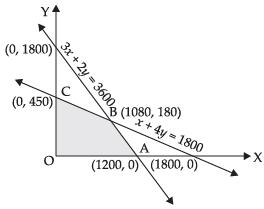Maximise Z = 100x + 170y subject to the constraints
3x + 2y ≤ 3600 ...(i)
x + 4y ≤ 1800 ...(ii)
x ≥ 0, y ≥ 0
On solving eq. (i) and (ii) we get
x = 1080 and y = 180
OABC is the feasible region whose corner points are O(0, 0),
A(1200, 0), B(1080, 180), C(0, 450).
Let us evaluate the value of Z.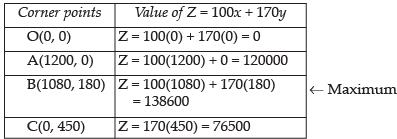Hence, the maximum value of Z is 138600 at (1080, 180).

Q.19. Refer to Exercise 14. How many sweaters of each type should the company make in a day to get a maximum profit? What is the maximum profit.
Ans.
Referring to the solution of Q. 14, we have
Maximise Z = 200x + 120y subject to the constraints
x + y ≤ 300    ...(i)
3x + y ≤ 600 ...(ii)
x - y ≥ - 100 ...(iii)
x ≥ 0, y ≥ 0
On solving eq. (i) and (iii) we have
x = 100, y = 200
On solving eq. (i) and (ii) we get
x = 150, y = 150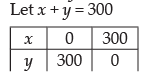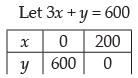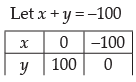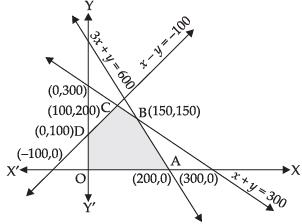Here, the shaded region is the feasible region whose corner points are O(0, 0), A(200, 0), B(150, 150), C(100, 200), D(0, 100).
Let us evaluate the value of Z.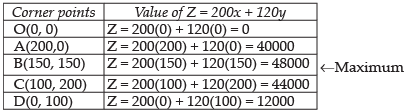Hence, the maximum value of Z is 48000 at (150, 150) i.e., 150 sweaters of each type.

Q.20. Refer to Exercise 15. Determine the maximum distance that the man can travel.
Ans.
Referring to the solution of Q. 15, we have
Maximise Z = x + y subject to the constraints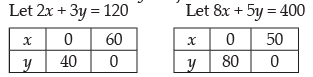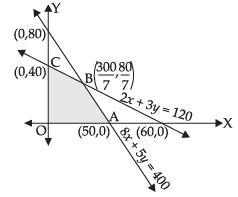2x + 3y ≤ 120 ...(i)
8x + 5y ≤ 400 ...(ii)
x ≥ 0, y ≥ 0
On solving eq. (i) and (ii) we get;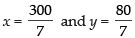Here, OABC is the feasible region whose corner points are O(0, 0), A(50, 0),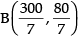and C(0, 40).
Let us evaluate the value of Z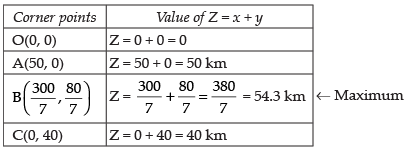Hence, the maximum distance that the man can travel is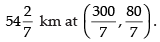Q.21. Maximise Z = x + y subject to x + 4y ≤ 8, 2x + 3y ≤ 12, 3x + y ≤ 9, x ≥ 0, y ≥ 0.
Ans.
We are given that Z = x + y subject to the constraints
x + 4y ≤ 8 ...(i)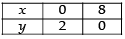2x + 3y ≤ 12 ...(ii)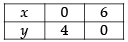3x + y ≤ 9 ...(iii)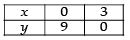x ≥ 0, y ≥ 0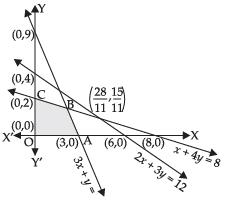On solving eq. (i) and (iii) we get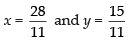Here, OABC is the feasible region whose corner points are
O(0, 0), A(3, 0),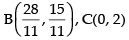Let us evaluate the value of Z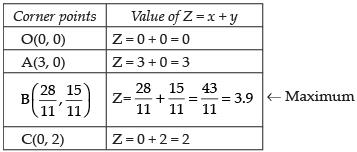Hence, the maximum value of Z is 3.9 atQ.22. A manufacturer produces two Models of bikes - Model X and Model Y. Model X takes a 6 man-hours to make per unit, while Model Y takes 10 man-hours per unit. There is a total of 450 man-hour available per week. Handling and Marketing costs are Rs 2000 and Rs 1000 per unit for Models X and Y respectively. The total funds available for these purposes are Rs 80,000 per week. Profits per unit for Models X and Y are Rs 1000 and Rs 500, respectively.
How many bikes of each model should the manufacturer produce so as to yield a maximum profit? Find the maximum profit.
Ans.
Let x  and y be the number of Models of bike produced by the manufacturer.
Given information is
Model X takes 6 man-hours to make per unit
Model Y takes 10 man-hours to make per unit
Total man-hours available = 450
∴  6x + 10y ≤ 450 ⇒ 3x + 5y ≤ 225   ...(i)
Handling and marketing cost of Model X and Y are ₹ 2,000 and ₹ 1,000 respectively
Total funds available is ₹ 80,000 per week
∴ 2000x + 1000y ≤ 80,000
⇒ 2x + y ≤ 80 ...(ii)
and x ≥ 80, y ≥ 0
Profit (Z) per unit of models X and Y are ₹ 1,000 and ₹ 500 respectively
So, Z = 1000x + 500y
The required LPP is Maximise Z = 1000x + 500y subject to the constraints
3x + 5y ≤ 225 ...(i)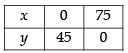2x + y ≤ 80 ...(ii)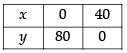x ≥ 0, y ≥ 0 ...(iii)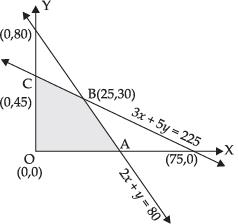On solving eq. (i) and (ii) we get, x = 25, y = 30
Here, the feasible region is OABC, whose corner points are
O(0, 0), A(40, 0), B(25, 30) and C(0, 45).
Let us evaluate the value of Z.Hence, the maximum profit is ₹ 40,000 by producing 25 bikes of Model X and 30 bikes of Model Y.

Q.23. In order to supplement daily diet, a person wishes to take some X and some wishes Y tablets. The contents of iron, calcium and vitamins in X and Y (in milligrams per tablet) are given as below: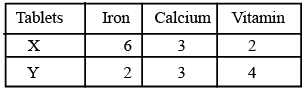The person needs at least 18 milligrams of iron, 21 milligrams of calcium and 16 milligram of vitamins. The price of each tablet of X and Y is Rs 2 and Re 1 respectively. How many tablets of each should the person take inorder to satisfy the above requirement at the minimum cost?
Ans.
Let there be x units of tablet X and y units of tablet Y
So, according to the given information, we have
6x + 2y ≥ 18 ⇒ 3x + y ≥ 9 ...(i)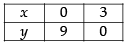3x + 3y ≥ 21 ⇒ x + y ≥ 7 ...(ii)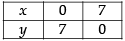2x + 4y ≥ 16 ⇒ x + 2y ≥ 8 ...(iii)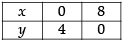x ≥ 0, y ≥ 0 ...(iv)
The price of each table of X type is ₹ 2 and that of y is ₹ 1.
So, the required LPP is
Minimise Z = 2x + y subject to the constraints
3x + y ≥ 9, x + y ≥ 7, x + 2y ≥ 8, x ≥ 0, y ≥ 0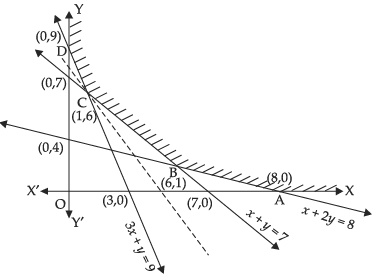On solving (ii) and (iii) we get
x = 6 and y = 1
On solving (i) and (ii) we get x = 1 and y = 6
From the graph, we see that the feasible region ABCD is unbounded whose corner points are A(8, 0), B(6, 1), C(1, 6) and D(0, 9).
Let us evaluate the value of Z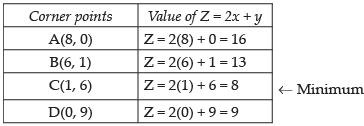Here, we see that 8 is the minimum value of Z at (1, 6) but the feasible region is unbounded. So, 8 may or may not be the minimum value of Z.
To confirm it, we will draw a graph of inequality 2x + y < 8 and check if it has a common point.
We see from the graph that there is no common point on the line.
Hence, the minimum value of Z is 8 at (1, 6).
Tablet X = 1
Table Y = 6.

Q.24. A company makes 3 model of calculators: A, B and C at factory I and factory II. The company has orders for at least 6400 calculators of model A, 4000 calculator of model B and 4800 calculator of model C. At factory I, 50 calculators of model A, 50 of model B and 30 of model C are made every day; at factory II, 40 calculators of model A, 20 of model B and 40 of model C are made everyday. It costs Rs 12000 and Rs 15000 each day to operate factory I and II, respectively. Find the number of days each factory should operate to minimise the operating costs and still meet the demand.
Ans.
Let factory I be operated for x days and II for y days
At factory I: 50 calculators of model A and at factory II, 40 calculators of model A are made everyday.
Company has orders of atleast 6400 calculators of model A.
∴ 50x + 40y ≥ 6400 ⇒ 5x + 4y ≥ 640
Also, at factory I, 50 calculators of model B and at factory II, 20 calculators of model B are made everyday.
Company has the orders of atleast 4000 of calculators of model B.
∴ 50x + 20y ≥ 4000
⇒ 5x + 2y ≥ 4000
Similarly for model C,
30x + 40y ≥ 4800
⇒ 3x + 4y ≥ 480
and x ≥ 0, y ≥ 0
It costs ₹ 12,000 and ₹ 15000 to operate the factories I and II each day.
∴ Required LPP is
Minimise Z = 12000x + 15000y subject to the constraints
5x + 4y ≥ 640 ...(i)
5x + 2y ≥ 400 ...(ii)
3x + 4y ≥ 480 ...(iii)
x ³ 0, y ≥ 0 ...(iv)
Table for (i) equation 5x + 4y = 640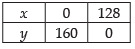Table for (ii) equation 5x + 2y = 400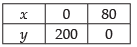Table for (iii) equation 3x + 4y = 480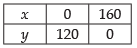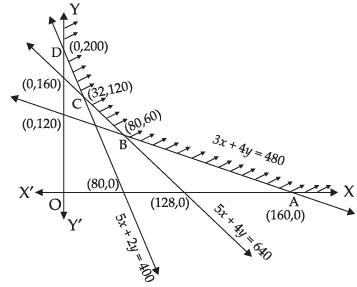On solving eq. (i) and (iii), we get
x = 80, y = 60
On solving eq. (i) and (ii) we get
x = 32 and y = 120
From the graph, we see that the feasible region ABCD is open unbounded whose corners are A(160, 0), B(80, 60), C(32, 120) and D(0, 200).
Let us find the values of Z.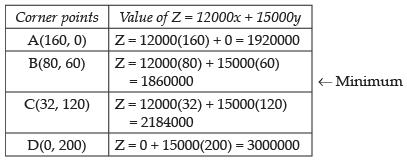From the above table, it is clear that the value of Z = 1860000
may or may not be minimum for an open unbounded region.
Now, to decide this, we draw a graph of
12000x + 15000y < 1860000
⇒ 4x + 5y < 620
and we have to check whether there is a common point in this feasible region or not.
So, from the graph, there is no common point.
Z = 12000x + 15000y has minimum value 1860000 at (80, 60).
Factory I : 80 days
Factory II: 60 days.

Q.25. Maximise and Minimise Z = 3x – 4y subject to
x – 2y ≤ 0
– 3x + y ≤ 4
x – y ≤  6
x, y ≥  0
Ans.
Given LPP is
Maximise and minimise Z = 3x – 4y subject to
x – 2y ≤ 0 ...(i)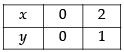– 3x + y ≤ 4 ...(ii)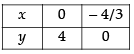x – y ≤ 6 ...(iii)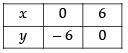and x, y ≥ 0 ...(iv)
From the graph, we see that AOB is open unbounded region whose corners are O(0, 0), A(0, 4), B(12, 6).
Let us evaluate the value of Z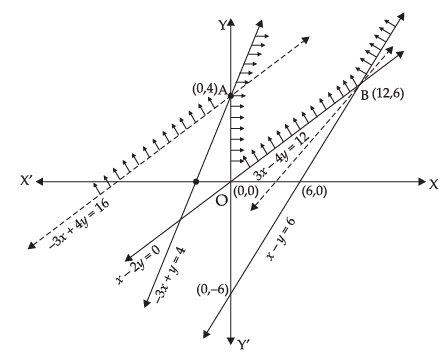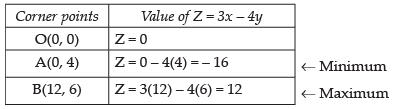For this unbounded region, the value of Z may or may not be – 16. So to decide it, we draw a graph of inequality 3x – 4y < – 16 and check whether the open half plane has common points with feasible region or not. But from the graph, we see that it has common points with the feasible region, so it will have not minimum value of Z. Similarly for maximum value, we draw the graph of inequality 3x – 4y > 12 in which there is no common point with the feasible region.
Hence, the maximum value of Z is 12.

OBJECTIVE TYPE QUESTIONS

Q.26. The corner points of the feasible region determined by the system of linear constraints are (0, 0), (0, 40), (20, 40), (60, 20), (60, 0). The objective function is Z = 4x + 3y. Compare the quantity in Column A and Column B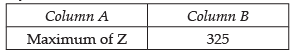(a) The quantity in column A is greater.
(b) The quantity in column B is greater
(c) The two quantities are equal
(d) The relationship cannot be determined on the basis of the
information supplied.
Ans. (b)
Solution.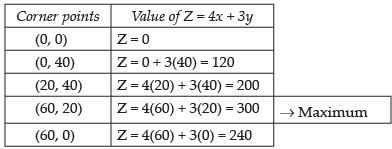Hence, the correct option is (b).

Q.27. The feasible solution for a LPP is shown in Figure. Let Z = 3x – 4y be the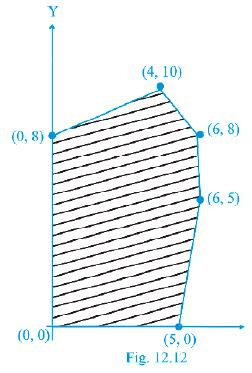objective function. Minimum of Z occurs at
(a) (0, 0)
(b) (0, 8)
(c) (5, 0)
(d) (4, 10)
Ans. (b)
Solution.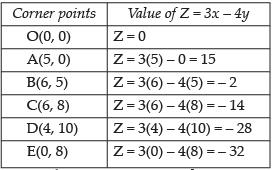← Minimum
Hence, the correct option is (b).

Q.28. Refer to Exercise 27. Maximum of Z occurs at

(a) (5, 0)
(b) (6, 5)
(c) (6, 8)
(d) (4, 10)
Ans. (a)
Solution.
According to solution of Q. 27, the maximum value of Z is 15 at A (5, 0).
Hence, the correct option is (a).

Q.29. Refer to Exercise 27. (Maximum value of Z + Minimum value of Z) is equal to
(a) 13
(b) 1
(c) – 13
(d) – 17
Ans. (d)
Solution.
According to the solution of Q. 27, Maximum value of Z = 15
and Minimum value of Z = – 32
So, the sum of Maximum value and Minimum value of Z
= 15 + (– 32) = – 17
Hence, the correct option is (d).

Q.30. The feasible region for an LPP is shown in the Figure. Let F = 3x – 4y be the objective function. Maximum value of F is.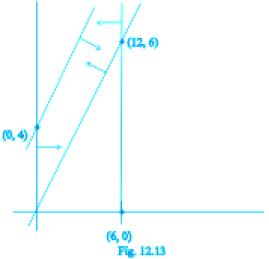(a) 0
(b) 8
(c) 12
(d) – 18
Ans. (c)
Solution.
The feasible region is shown in the figure for which the objective function F = 3x – 4y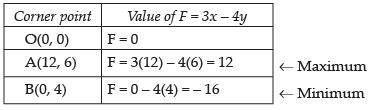Hence, the correct option is (c).

Q.31. Refer to Exercise 30. Minimum value of F is
(a) 0
(b) – 16
(c) 12
(d) does not exist
Ans. (b)
Solution.
According to the solution of Q. 30, the minimum value of F is – 16 at (0, 4).
Hence, the correct option is (b).

Q.32. Corner points of the feasible region for an LPP are (0, 2), (3, 0), (6, 0), (6, 8) and (0, 5). Let F = 4x + 6y be the objective function.
The Minimum value of F occurs at
(a) (0, 2) only
(b) (3, 0) only
(c) the mid-point of the line segment joining the points (0, 2) and (3, 0) only
(d) any point on the line segment joining the points (0, 2) and (3, 0)
Ans. (d)
Solution.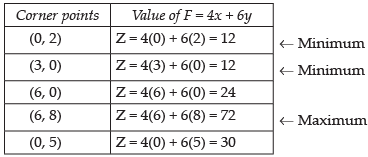The minimum value of F occurs at any point on the line segment joining the points (0, 2) and (3, 0).
Hence, the correct option is (d).

Q.33. Refer to Exercise 32, Maximum of F – Minimum of F =
(a) 60
(b) 48
(c) 42
(d) 18
Ans. (a)
Solution.
According to the solution of Q. 32, Maximum value of F – Minimum value of F = 72 – 12 = 60
Hence, the correct option is (a).

Q.34. Corner points of the feasible region determined by the system of linear constraints are (0, 3), (1, 1) and (3, 0). Let Z = px+qy, where p, q > 0. Condition on p and q so that the minimum of Z occurs at (3, 0) and (1, 1) is
(a) p = 2q
(b) p = q/2
(c) p = 3q
(d) p = q
Ans. (b)
Solution.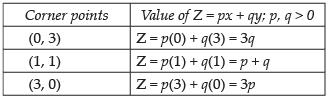So, condition of p and q so that the minimum of Z occurs at (3, 0) and (1, 1) is
p + q = 3p
⇒ p – 3p + q = 0
⇒ p =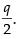Hence, the correct option is (b).

Fill in the blanks
Q.35. In a LPP, the linear inequalities or restrictions on the variables are called _________.
Ans. constraints.

Q.36. In a LPP, the objective function is always _________.
Ans. linear

Q.37. If the feasible region for a LPP is _________, then the optimal value of the objective function Z = ax + by may or may not exist.
Ans. open unbounded

Q.38. In a LPP if the objective function Z = ax + by has the same maximum value on two corner points of the feasible region, then every point on the line segment joining these two points give the same _________ value.
Ans. maximum

Q.39. A feasible region of a system of linear inequalities is said to be _________ if it can be enclosed within a circle.
Ans. bounded

Q.40. A corner point of a feasible region is a point in the region which is the _________ of two boundary lines.
Ans. intersection

Q.41. The feasible region for an LPP is always a _________ polygon.
Ans. convex

State whether the statements in Exercises 42 to 45 are True or False.
Q.42. If the feasible region for a LPP is unbounded,  maximum or minimum of the objective function Z = ax + by may or may not exist.
Ans. True

Q.43. Maximum value of the objective function Z = ax + by in a LPP always occurs at only one corner point of the feasible region.
Ans. False

Q.44. In a LPP, the minimum value of the objective function Z = ax + by is always 0 if origin is one of the corner point of the feasible region.
Ans. False

Q.45. In a LPP, the maximum value of the objective function Z = ax + by is always finite.
Ans. True

Offer running on EduRev: Apply code STAYHOME200 to get INR 200 off on our premium plan EduRev Infinity!

## Mathematics (Maths) Class 12

209 videos|222 docs|124 tests

,

,

,

,

,

,

,

,

,

,

,

,

,

,

,

,

,

,

,

,

,

;